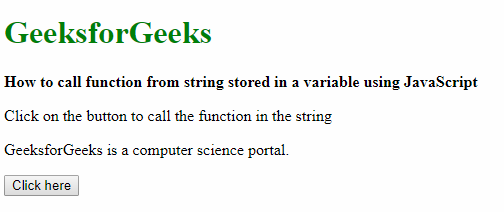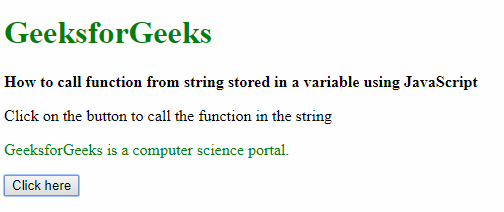Related Articles

# How to call function from it name stored in a string using JavaScript?

• Difficulty Level : Easy
• Last Updated : 21 Apr, 2019

There are two methods to call a function from string stored in a variable. The first one is by using the window object method and the second one is by using eval() method. The eval() method is older and it is deprecated.

Method 1: Using the window object: The window object in HTML 5 references the current window and all items contained in it. Hence we can use it to run a function in a string along with parameters to the function.

Syntax:

`window[functionName](parameters)`

Example:

 ```<``html``>``     ` `<``head``>``    ``<``title``>``        ``How to call function from string stored``        ``in a variable using JavaScript``    `````` ` `<``body``>``    ``<``h1` `style``=``"color: green"``>``        ``GeeksforGeeks``    ````     ` `    ``<``b``>``        ``How to call function from string stored``        ``in a variable using JavaScript ``    ````     ` `    ``<``p``>``        ``Click on the button to call the``        ``function in the string``    ````     ` `    ``<``p` `class``=``"example"``>``        ``GeeksforGeeks is a computer``        ``science portal.``    ```` ` `    ``<``button` `onclick``=``"evaluateFunction()"``>``        ``Click here``    ````     ` `    ``<``script` `type``=``"text/javascript"``>``     ` `        ``function changeColor(color) {``            ``document.querySelector('.example').style``                ``= `color: \${color}`;``        ``}`` ` `        ``function evaluateFunction() {``            ``stringFunction = "changeColor";``            ``param = 'green';``            ``window[stringFunction](param);``        ``}``    `````` ` `                    `

Output:

• Before clicking the button:• After clicking the button:Method 2: Using the eval() method: The other method that can be used is the eval() method. The string that may be passed on to the function may include a JavaScript expression, statement, or a sequence of statements. It can also have variables and properties of existing objects. The only problem with this method is that it is considered unsafe and may not be supported by newer browsers.

Syntax:

`eval(stringFunction)`

Example:

 ```<``html``>``     ` `<``head``>``    ``<``title``>``        ``How to call function from string stored``        ``in a variable using JavaScript``    `````` ` `<``body``>``    ``<``h1` `style``=``"color: green"``>``        ``GeeksforGeeks``    ````     ` `    ``<``b``>``        ``How to call function from string stored``        ``in a variable using JavaScript ``    ````     ` `    ``<``p``>``        ``Click on the button to call the``        ``function in the string``    ````     ` `    ``<``p` `class``=``"example"``>``        ``GeeksforGeeks is a computer``        ``science portal.``    ```` ` `    ``<``button` `onclick``=``"evaluateFunction()"``>``        ``Click here``    ````     ` `    ``<``script` `type``=``"text/javascript"``>``     ` `        ``function changeColor(color) {``            ``document.querySelector('.example').style``                    ``= `color: \${color}`;``        ``}``     ` `        ``function evaluateFunction() {``            ``stringFunction = "changeColor('green')";``            ``eval(stringFunction);``        ``}``    `````` ` `                    `

Output:

• Before clicking the button:• After clicking the button:My Personal Notes arrow_drop_up